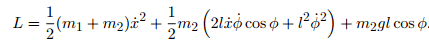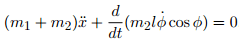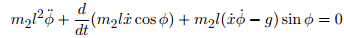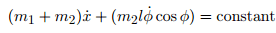# Conserved quantities in the cart and pendulum problem

A problem on an assignment I'm doing deals with a cart of mass m1 which can slide frictionlessly along the x-axis. Suspended from the cart by a string of length l is a mass m2, which is constrained to move in the x-y plane. The angle between the pendulum and vertical is notated as phi. The question asks me to first derive the Lagrangian for the system which I got as:It then asks me to find any continuous symmetrys in the problem and the corresponding conserved quantities. I found the Euler-Lagrange equations for x:and for phi:From the E-L equation for x, I know that:Which I believe indicates the momentum in the x-direction is conserved, but I'm not sure. I think I'm supposed to do this with some application of Noether theorem, which was supposed to be covered today, but class was cancelled due to snow and the assignment is still due Wednesday. Can anyone help me?

BvU